|

# 看欧洲精品瓷砖在2017博洛尼亚展上怎么“玩”的?

2017 博洛尼亚陶瓷卫浴展正在如火如荼的进行当中，来自世界各地的相关企业在这个陶瓷及卫浴领域内规模最大专业度最高的展会上争奇斗艳，通过博洛尼亚展的舞台将品牌最新的产品、设计理念、设备技术、用户体验等等展现给全世界。MYHOME 明禾吉利旗下三大欧洲精品瓷砖品牌也在展会上完成了各自的亮相，每个品牌都引起了极大的关注度与讨论度。下面跟随我们一起走进 2017 博洛尼亚陶瓷卫浴展，感受最前沿的陶瓷产品与最潮流的家居文化。西班牙瓷砖品牌 PAMESA 今年的展厅外观设计可谓中规中矩，虽然展厅外围采用极简的设计，但是展厅内部可谓别有洞天。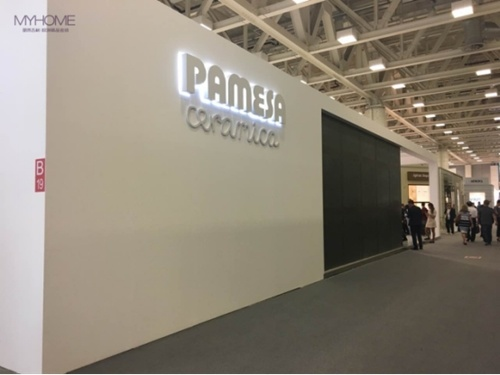展厅门口负责接待的西班牙美女热情奔放，对于观众的咨询有问必答，这与PAMESA品牌富有活力与激情的品牌调性不谋而合。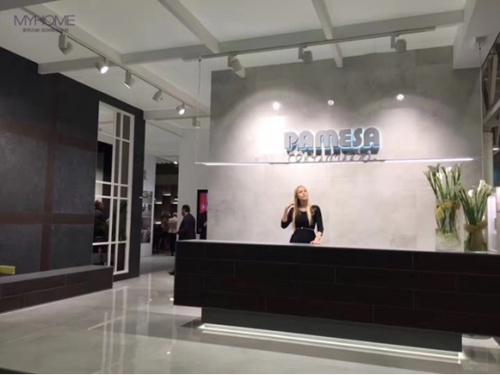在中国市场上极受欢迎的水泥面瓷砖 K-KONCEPT 极简系列，将全系列产品上墙展示，方便消费者直观的欣赏与对比。其中 K-WOOD 多款新品将水泥原始的质感与树木自然的纹路相结合令人眼前一亮。在大规格瓷砖大行其道的年代，PAMESA 也带来了多款 120×3000px 大规格现代化方砖新品。PAMESA 除了传统的产品展示墙外，还将瓷砖结合丰富多样的软装打造真实的家居空间，让消费者对产品铺贴后的实际效果一目了然。如果这些样板间满足不了你对家的想象，那么还可以去 PAMESA 展厅内的 VR 虚拟体验区，通过高科技手段感受身临其境的装修效果。通过触屏的方式选择瓷砖风格、纹理、颜色、规格等信息，然后戴上 PAMESA 与专业科技公司合作的 VR 眼镜，一个经你设计完成的虚拟环绕家居空间将呈现在眼前。PAMESA 展厅内的艺术专区人潮涌动，来自世界各地的艺术家、画家、设计师等在这里展示他们的作品并进行交流分享。PAMESA 对于艺术与瓷砖的辩证关系是这样定义的“释放你的创造力，通过木头与水泥的纹理质感创造符合品味的艺术品。”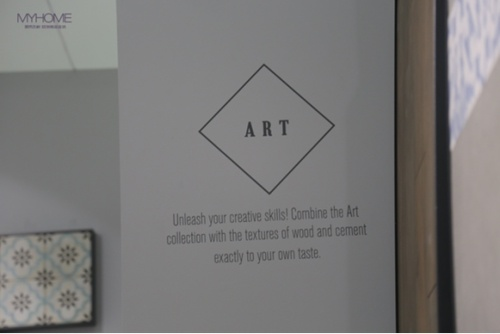与 PAMESA 公司保持长期合作关系的西班牙跨界设计师阿加莎也带了她所设计的产品，依然以艳丽缤纷的颜色与充满童趣的图案表现强烈的个人风格。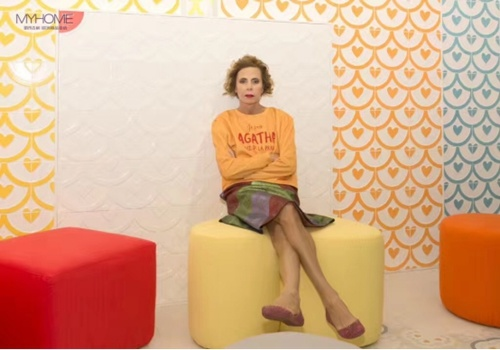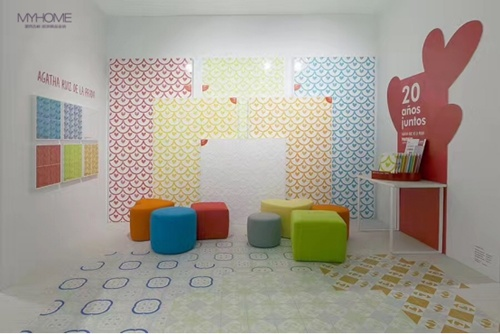意大利 ABK 瓷砖展厅门口三块超大的 LED 屏滚动播放着品牌宣传片，炫酷的光影效果吸引大批人潮。ABK 公司在今年展会上的阵容规模空前强大，以至于搭建了两个展厅用来展示自家的最新产品和企业文化，展位面积之大，也是历届展会前所未有的。与展厅外科技感十足的灯光秀相反，ABK 展厅内布置成庭院悠悠的绿色空间。作为欧洲精品瓷砖四大主流中的水泥面“砖”家，ABK 带来了多款大板水泥面瓷砖新品，通过信息表可以看出同一款瓷砖，能够集结多种不同风格的色彩与质感。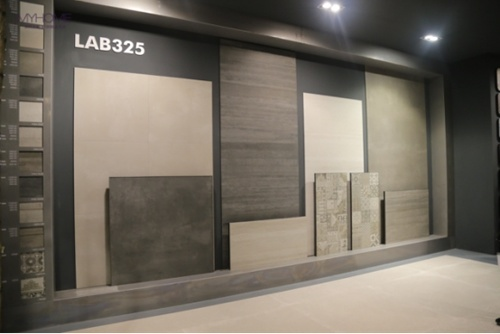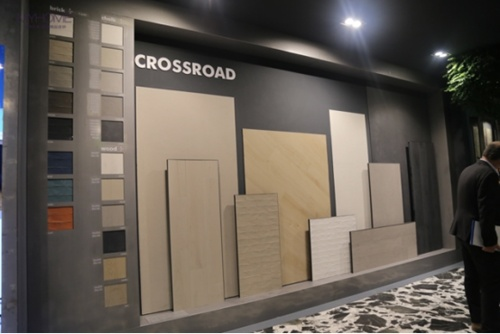在中国市场深受消费者喜爱的生态石产品 SENIS 灵感石系列也展示多款大板新品，纹路更加细腻自然，亮度色泽饱满通透，大气时尚之美油然而生。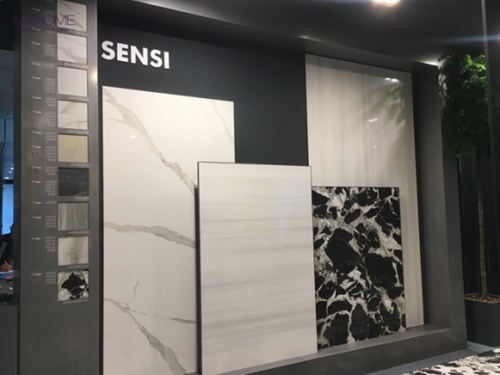作为唯一专注表面设计的瓷砖品牌，ABK 在瓷砖表面的艺术境界已经达到了登峰造极的地步。下面这幅栩栩如生的油画作品其实是由一整块大规格瓷砖制作而成，这样兼具艺术与实用的作品完全可以取代传统壁纸作背景墙使用。ABK 将展厅打造成陶瓷的海洋，橱柜、餐桌等家具全部采用陶瓷制作，充满艺术感的陶瓷家具令人叹为观止。随着时代的发展与进步，“陶瓷正在打破传统形式成为新的材料”，工艺和设计的发展使陶瓷在未来不断变化，应用于更多领域。ABK 还在展厅内布置了许多具有年代感的物品，这些别具一格的装饰品向世界传递着意大利悠久的历史文化与精致的生活方式。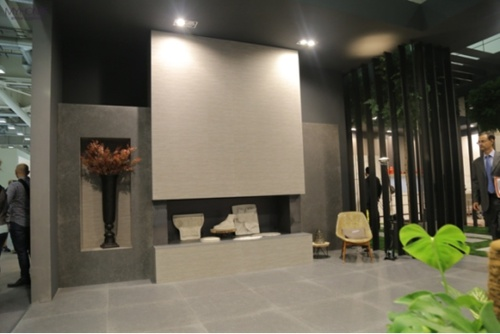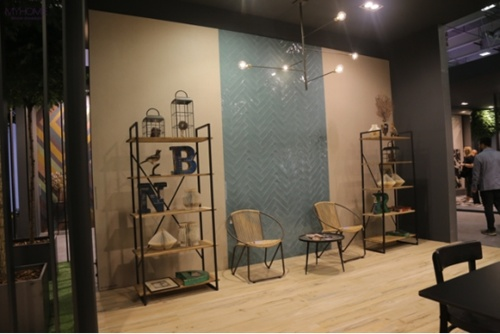意大利瓷砖品牌 Rondine 的展位面积比去年大了 200 平方米达到 600 平方米。Rondine 将整个展区打造成了绿色的海洋，从外观看如果不了解这是一家陶企的展位还以为是园博会呢。这样绿色生态的展区环境，也彰显了 Rondine 公司一直坚守自然、健康、环保的企业理念。 “Rondine is green” 的口号始终贯穿于 Rondine 展区的各个角落。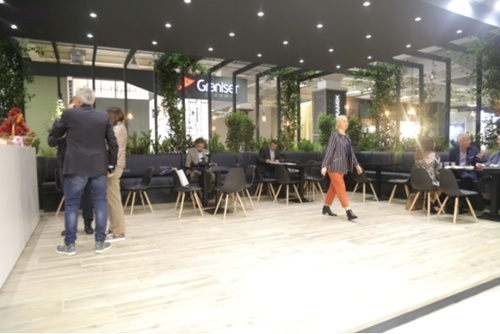Rondine 公司被称为“墙面艺术大师”，善于通过对其他材质的模仿达到“以假乱真”的效果。最新的 3d 墙砖产品，通过技术手段达到在同一块砖表面展现不同厚度的立体效果。近些年，祛繁从简、返璞归真的审美标准深入人心，这让水泥面与原木木纹的的装饰效果大放异彩。Rondine 水泥面系列瓷砖以不同层次的灰诠释现代简约美学。复古系列原木木纹砖，亮度、纹理与铺贴方式完美保留了令人回味的复古韵味。

关于我们

MYHOME明禾吉利-欧洲精品瓷砖，1996年成立于北京，专营原装进口、原厂品牌欧洲精品瓷砖。MYHOME明禾吉利以“欧洲同步 原版体验”为经营理念，旗下独立合作品牌有西班牙PAMESA瓷砖、意大利ABK瓷砖，意大利RONDINE瓷砖等，并相继获得授权成立中国区代表处。展示中心所有产品均是100%原装进口的原厂品牌产品，拥有全球大理石瓷砖、生态石瓷砖、原木木纹瓷砖、水泥面瓷砖四大主流趋势产品的领导地位。

`声明：本文由入驻焦点开放平台的作者撰写，除焦点官方账号外，观点仅代表作者本人，不代表焦点立场错误信息举报电话： 400-099-0099，邮箱：jubao@vip.sohu.com，或点此进行意见反馈，或点此进行举报投诉。`A B C D E F G H J K L M N P Q R S T W X Y Z
A - B - C - D - E
• A
• 鞍山
• 安庆
• 安阳
• 安顺
• 安康
• 澳门
• B
• 北京
• 保定
• 包头
• 巴彦淖尔
• 本溪
• 蚌埠
• 亳州
• 滨州
• 北海
• 百色
• 巴中
• 毕节
• 保山
• 宝鸡
• 白银
• 巴州
• C
• 承德
• 沧州
• 长治
• 赤峰
• 朝阳
• 长春
• 常州
• 滁州
• 池州
• 长沙
• 常德
• 郴州
• 潮州
• 崇左
• 重庆
• 成都
• 楚雄
• 昌都
• 慈溪
• 常熟
• D
• 大同
• 大连
• 丹东
• 大庆
• 东营
• 德州
• 东莞
• 德阳
• 达州
• 大理
• 德宏
• 定西
• 儋州
• 东平
• E
• 鄂尔多斯
• 鄂州
• 恩施
F - G - H - I - J
• F
• 抚顺
• 阜新
• 阜阳
• 福州
• 抚州
• 佛山
• 防城港
• G
• 赣州
• 广州
• 桂林
• 贵港
• 广元
• 广安
• 贵阳
• 固原
• H
• 邯郸
• 衡水
• 呼和浩特
• 呼伦贝尔
• 葫芦岛
• 哈尔滨
• 黑河
• 淮安
• 杭州
• 湖州
• 合肥
• 淮南
• 淮北
• 黄山
• 菏泽
• 鹤壁
• 黄石
• 黄冈
• 衡阳
• 怀化
• 惠州
• 河源
• 贺州
• 河池
• 海口
• 红河
• 汉中
• 海东
• 怀来
• I
• J
• 晋中
• 锦州
• 吉林
• 鸡西
• 佳木斯
• 嘉兴
• 金华
• 景德镇
• 九江
• 吉安
• 济南
• 济宁
• 焦作
• 荆门
• 荆州
• 江门
• 揭阳
• 金昌
• 酒泉
• 嘉峪关
K - L - M - N - P
• K
• 开封
• 昆明
• 昆山
• L
• 廊坊
• 临汾
• 辽阳
• 连云港
• 丽水
• 六安
• 龙岩
• 莱芜
• 临沂
• 聊城
• 洛阳
• 漯河
• 娄底
• 柳州
• 来宾
• 泸州
• 乐山
• 六盘水
• 丽江
• 临沧
• 拉萨
• 林芝
• 兰州
• 陇南
• M
• 牡丹江
• 马鞍山
• 茂名
• 梅州
• 绵阳
• 眉山
• N
• 南京
• 南通
• 宁波
• 南平
• 宁德
• 南昌
• 南阳
• 南宁
• 内江
• 南充
• P
• 盘锦
• 莆田
• 平顶山
• 濮阳
• 攀枝花
• 普洱
• 平凉
Q - R - S - T - W
• Q
• 秦皇岛
• 齐齐哈尔
• 衢州
• 泉州
• 青岛
• 清远
• 钦州
• 黔南
• 曲靖
• 庆阳
• R
• 日照
• 日喀则
• S
• 石家庄
• 沈阳
• 双鸭山
• 绥化
• 上海
• 苏州
• 宿迁
• 绍兴
• 宿州
• 三明
• 上饶
• 三门峡
• 商丘
• 十堰
• 随州
• 邵阳
• 韶关
• 深圳
• 汕头
• 汕尾
• 三亚
• 三沙
• 遂宁
• 山南
• 商洛
• 石嘴山
• T
• 天津
• 唐山
• 太原
• 通辽
• 铁岭
• 泰州
• 台州
• 铜陵
• 泰安
• 铜仁
• 铜川
• 天水
• 天门
• W
• 乌海
• 乌兰察布
• 无锡
• 温州
• 芜湖
• 潍坊
• 威海
• 武汉
• 梧州
• 渭南
• 武威
• 吴忠
• 乌鲁木齐
X - Y - Z
• X
• 邢台
• 徐州
• 宣城
• 厦门
• 新乡
• 许昌
• 信阳
• 襄阳
• 孝感
• 咸宁
• 湘潭
• 湘西
• 西双版纳
• 西安
• 咸阳
• 西宁
• 仙桃
• 西昌
• Y
• 运城
• 营口
• 盐城
• 扬州
• 鹰潭
• 宜春
• 烟台
• 宜昌
• 岳阳
• 益阳
• 永州
• 阳江
• 云浮
• 玉林
• 宜宾
• 雅安
• 玉溪
• 延安
• 榆林
• 银川
• Z
• 张家口
• 镇江
• 舟山
• 漳州
• 淄博
• 枣庄
• 郑州
• 周口
• 驻马店
• 株洲
• 张家界
• 珠海
• 湛江
• 肇庆
• 中山
• 自贡
• 资阳
• 遵义
• 昭通
• 张掖
• 中卫

1室1厅1厨1卫1阳台

1
2
3
4
5

0
1
2

1

1

0
1
2
3报名成功，资料已提交审核A B C D E F G H J K L M N P Q R S T W X Y Z
A - B - C - D - E
• A
• 鞍山
• 安庆
• 安阳
• 安顺
• 安康
• 澳门
• B
• 北京
• 保定
• 包头
• 巴彦淖尔
• 本溪
• 蚌埠
• 亳州
• 滨州
• 北海
• 百色
• 巴中
• 毕节
• 保山
• 宝鸡
• 白银
• 巴州
• C
• 承德
• 沧州
• 长治
• 赤峰
• 朝阳
• 长春
• 常州
• 滁州
• 池州
• 长沙
• 常德
• 郴州
• 潮州
• 崇左
• 重庆
• 成都
• 楚雄
• 昌都
• 慈溪
• 常熟
• D
• 大同
• 大连
• 丹东
• 大庆
• 东营
• 德州
• 东莞
• 德阳
• 达州
• 大理
• 德宏
• 定西
• 儋州
• 东平
• E
• 鄂尔多斯
• 鄂州
• 恩施
F - G - H - I - J
• F
• 抚顺
• 阜新
• 阜阳
• 福州
• 抚州
• 佛山
• 防城港
• G
• 赣州
• 广州
• 桂林
• 贵港
• 广元
• 广安
• 贵阳
• 固原
• H
• 邯郸
• 衡水
• 呼和浩特
• 呼伦贝尔
• 葫芦岛
• 哈尔滨
• 黑河
• 淮安
• 杭州
• 湖州
• 合肥
• 淮南
• 淮北
• 黄山
• 菏泽
• 鹤壁
• 黄石
• 黄冈
• 衡阳
• 怀化
• 惠州
• 河源
• 贺州
• 河池
• 海口
• 红河
• 汉中
• 海东
• 怀来
• I
• J
• 晋中
• 锦州
• 吉林
• 鸡西
• 佳木斯
• 嘉兴
• 金华
• 景德镇
• 九江
• 吉安
• 济南
• 济宁
• 焦作
• 荆门
• 荆州
• 江门
• 揭阳
• 金昌
• 酒泉
• 嘉峪关
K - L - M - N - P
• K
• 开封
• 昆明
• 昆山
• L
• 廊坊
• 临汾
• 辽阳
• 连云港
• 丽水
• 六安
• 龙岩
• 莱芜
• 临沂
• 聊城
• 洛阳
• 漯河
• 娄底
• 柳州
• 来宾
• 泸州
• 乐山
• 六盘水
• 丽江
• 临沧
• 拉萨
• 林芝
• 兰州
• 陇南
• M
• 牡丹江
• 马鞍山
• 茂名
• 梅州
• 绵阳
• 眉山
• N
• 南京
• 南通
• 宁波
• 南平
• 宁德
• 南昌
• 南阳
• 南宁
• 内江
• 南充
• P
• 盘锦
• 莆田
• 平顶山
• 濮阳
• 攀枝花
• 普洱
• 平凉
Q - R - S - T - W
• Q
• 秦皇岛
• 齐齐哈尔
• 衢州
• 泉州
• 青岛
• 清远
• 钦州
• 黔南
• 曲靖
• 庆阳
• R
• 日照
• 日喀则
• S
• 石家庄
• 沈阳
• 双鸭山
• 绥化
• 上海
• 苏州
• 宿迁
• 绍兴
• 宿州
• 三明
• 上饶
• 三门峡
• 商丘
• 十堰
• 随州
• 邵阳
• 韶关
• 深圳
• 汕头
• 汕尾
• 三亚
• 三沙
• 遂宁
• 山南
• 商洛
• 石嘴山
• T
• 天津
• 唐山
• 太原
• 通辽
• 铁岭
• 泰州
• 台州
• 铜陵
• 泰安
• 铜仁
• 铜川
• 天水
• 天门
• W
• 乌海
• 乌兰察布
• 无锡
• 温州
• 芜湖
• 潍坊
• 威海
• 武汉
• 梧州
• 渭南
• 武威
• 吴忠
• 乌鲁木齐
X - Y - Z
• X
• 邢台
• 徐州
• 宣城
• 厦门
• 新乡
• 许昌
• 信阳
• 襄阳
• 孝感
• 咸宁
• 湘潭
• 湘西
• 西双版纳
• 西安
• 咸阳
• 西宁
• 仙桃
• 西昌
• Y
• 运城
• 营口
• 盐城
• 扬州
• 鹰潭
• 宜春
• 烟台
• 宜昌
• 岳阳
• 益阳
• 永州
• 阳江
• 云浮
• 玉林
• 宜宾
• 雅安
• 玉溪
• 延安
• 榆林
• 银川
• Z
• 张家口
• 镇江
• 舟山
• 漳州
• 淄博
• 枣庄
• 郑州
• 周口
• 驻马店
• 株洲
• 张家界
• 珠海
• 湛江
• 肇庆
• 中山
• 自贡
• 资阳
• 遵义
• 昭通
• 张掖
• 中卫• 手机• 分享
• 设计
免费设计
• 计算器
装修计算器
• 入驻
合作入驻
• 联系
联系我们
• 置顶
返回顶部